# Natural Physical Length Contraction Due to Gravity

### Paul Marmet (1932-2005)

( Last checked 2018/01/16 - The estate of Paul Marmet )
Return to: List of Papers on the Web
Go to: Frequently Asked Questions

Eine deutsche Übersetzung dieses Artikels finden Sie hier.

Abstract.
This paper demonstrates how quantum mechanics solves the problem previously attributed to relativity, when atoms acquire gravitational energy.  When we apply the principle of mass-energy conservation to an atom gaining potential energy, the electron mass increases.  Then, using the fundamental principles of quantum mechanics, we show that the Bohr radius decreases so that the physical macroscopic length of bodies also decreases.  Classical physics with quantum mechanics alone lead to predictions, which are compatible with all the experimental observations usually attributed to relativity.  It has been shown previously how the increase of kinetic energy changes the atomic structure, due to the change of electron mass.  We explain here the corresponding phenomenon, when the increase of electron mass is due to gravitational energy.  Such an increase of potential energy leads to an increase of frequency of emission of atoms in agreement with Pound and Rebka experiment.  This paper also explains the combined physical phenomena, taking place when an atom increases its kinetic energy at the same time as an increase of potential energy.  We see then, how the physical length of matter changes and how the natural rate of clocks, change in such a way that it explains naturally the advance of the perihelion of Mercury.  Furthermore, we show how the increase of potential energy leads to a different change of Bohr radius, than when an increase of kinetic energy is involved.  This dissimilarity is due to the difference of momentum transfer during the interaction of kinetic and potential energy.  This is explained naturally, without any need of Einstein's theory of relativity.  Conventional logic and realistic physics are now sufficient to explain all these phenomena in nature.  The esoteric hypothesis of space and time distortion is useless.  Instead, using realism, we can understand how the physical change of length of bodies and clock rates, take place in a gravitational field.

1-- Introduction.
There exists no physical rationalization explaining why and how matter can dilate or contract as claimed in relativity.  That physics theory is impenetrable, because it is not compatible with the existence of an absolute physical reality, independent of the observer.  Einstein's theory has never been able to provide a logical description of the physical meaning of relativity.  Unfortunately, just as during the Middle Ages, most scientists claim that nature is not compatible with conventional logic.  Magic is required in the interpretation of Einstein's relativity.
In this paper, the phenomenon of length contraction or dilation of matter and the change of clock rate are explained logically without any of Einstein's relativity hypotheses (1).  We have seen in a previous paper (2-4) how a simple classical mechanism can explain the physical length contraction and dilation of matter, when the mass of the proton and of the electron inside the atom increases due to the absorption of kinetic energy.  As expected, a comparable increase of electron and proton masses occurs, due to the addition of gravitational potential energy.  Due to the variation of potential energy and the application of the principle of mass-energy conservation, we show how the Bohr radius increases and decreases, so that the physical size of matter changes.  Dilation of matter is not a simple mathematical transformation: it is a physical reality.  Also, matter shrinks back to its original length when the atom returns to the original potential energy as explained previously (2,3)
Here, we show with full details, how matter is dilated and contracted, and how clocks run at a different rate due to the change of gravitational potential energy.  Following a change of quantum structure, we can see how that change of gravitational potential energy is responsible for the change of physical size of the atom.  Contrary to most papers in modern physics, we always refer to a realistic physical model.  In contrast to Einstein's relativity, we show that all the phenomena previously claimed to belong to relativity, can now be described using models of the kind used by Newton, Coulomb and de Broglie.  The importance of the de Broglie wavelength relationship is vital.  The reader must be able to conceive first that, due to the potential energy of atoms, the electron mass increases.  Furthermore, there is a change of size of the "reference units" in different frames, which is a direct consequence of the principle of mass-energy conservation.
It is also an experimental fact that when an electric charge (i. e. an electron) is accelerated to high velocity, its mass increases, but its electric charge remains constant.  This is verified experimentally when an electron is accelerated to velocities close to the velocity of light, and then deflected in a magnetic field.  Experimental data show clearly that, the electron charge-to-mass ratio (e/m) changes with velocity in such a way (just as expected), that the electron mass increases as a function of velocity, while the electric charge remains constant at any velocity.  This is a well-verified experimental fact.  Therefore, just as in the case of kinetic energy, in a gravitational potential, the electron mass increases with potential energy and the electric charge remains constant.
We recall that an accelerating electric charge generates electromagnetic radiation.  Consequently, a quantum transition inside a particle always emits a quantized amount of energy.  In some cases, that flow of electromagnetic radiation can be continuous, but its detection is quantized due to the quantum states of atoms and molecules in the detector.
In this paper, we consider separately the influence of the gravitational potential on atoms, assuming momentarily that the kinetic energy is maintained at zero.  The problem of kinetic energy has been calculated in a previous paper (5) and does not need to be reconsidered here.  The effects of kinetic and potential energy on atoms are considered independently before their physical effect are finally combined in the last section of this paper.  Let us calculate first how the atomic structure of the atoms changes as a function of the gravitational potential in which they are immerged.

2-- Mass-Energy Conservation in Gravitational Potential.
We have seen previously (2, 3) that when a body is moved between different altitudes in a gravitational field, its mass must change in order to be compatible with the principle of mass-energy conservation.  Let us recall the following experiment.  We consider that an individual hydrogen atom is located at its initial distance yo from a gravitational source. This is illustrated on Figure 1.Figure 1
The distance yo is the radial distance between the center of the gravitational field and the mass mo of the particle under study. At that location, the gravitational acceleration is g.  The direction of the gravitational acceleration g is negative, because the gravitational force is directed toward the center of mass.  A hydrogen atom is attached to a fine weightless thread, so that the atom can be lowered down slowly across the distance "minus Dy" in the gravitational field, while the experimenter (with his measuring instruments) remains at location yo.  In the gravitational field, the weight of mo produces a force F in the thread.  That force, measured at the original location yo (using the units of location yo) is:
F = mo g.           1
The slow descent of the atom attached to the thread is stopped every time a measurement is made.  Therefore, the kinetic energy is always zero during all measurements.  When the atom has traveled a radial distance -Dy, the atom is closer to the center of the gravitational field and the observer measures that the force F on the atom, has produced an energy DE, transmitted through the thread to the original location yo.  The energy DE transmitted is:
DE = F Dy.           2
In equation 2, we note that, when the mass gets lower, both F and Dy are negative.  Then, the amount of energy DE transmitted through the tread to location yo is positive.  Using the principle of mass-energy conservation, let us calculate the extra mass Dm, which is generated at the observer's location yo, due to the arrival of the energy DE, as a consequence of the descent.  We have:
DE = Dm c2           3
The fundamental point in equation 3 is that the energy DE is proportional to the extra mass Dm generated.  The use of equation E=mc2 has been explained previously (2) (equation 2.3). The parameter c2 is the constant of proportionality between mass (kilogram) and energy (joule).   Therefore, when there is a change of gravitational energy between locations yo and y(-Dy), this leads to a resultant change of mass Dm corresponding to DE.  From equations 1, 2 and 3, the amount of mass Dmo generated by the force on the tread (using the units existing at the original location yo), due to the descent of the particle across the distance Dy is:4
Due to the descent of the mass across the distance Dy, an energy DE (therefore a mass Dmo carried by the string) appears at location yo. Applying the principle of mass-energy conservation, we find that the same amount of mass (Dm(-Dy)) must disappears at the lower location y(-Dy), than the amount created Dmo, at location yo, where the energy has been moved.  We have:5
Therefore the mass Dmo, lost due to the transfer of the energy through the thread, moving from y(-Dy) to yo must be subtracted from mo.  Using equation 4, the mass m(-Dy) at location y(-Dy) is: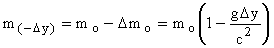6
In the equations above, Dy is negative when the mass moves down.  The gravitational acceleration g, which is always directed downward, is also negative.  It is important to recall that the masses above must always be calculated using the units existing in the initial frame located at yo.  Using the principle of mass-energy conservation, the mass of the hydrogen atom at location y(-Dy), is now smaller than its initial mass mo when it was at the original location yo.  Any variation of g with height is irrelevant, because the exact value of g is taken into account in equations 4 and 6.  In fact, we consider in equation 6, that the change of energy DE (and therefore Dm) is always the integral of the local gravitational force g, times the distance Dy.  Of course, since the change of mass Dmo is so small, the relative change of mass (Dmo/mo) (which is 10-15 in Pound and Rebka experiment (6)) is an extremely small fraction.  In this paper, for practical reasons, we consider the absolute value of |g|, which is always positive.  We have:7
Also, for practical reasons, we define a new variable e as:8
Let us now consider a positive increase of distance +Dy from the gravitational source.  Equations 6, 7 and 8 give:9

In compatibility with the definition above, we recall that m+Dy is the mass at a location +Dy above the original location at yo.  We have seen previously (2) that equation 9 is compatible with experimental data.  This calculated change of energy levels as a function of gravitational potential is in perfect agreement with the Pound and Rebka and also the Pound and Snider experiments (6).  They used Mössbauer spectroscopy to measure the red shift of 14.4 keV gamma rays from Fe57.  In those experiments, the emitter and the absorber were placed at rest, at the bottom and the top of a 22.5 meters high tower at Harvard University.  Pound and Rebka reported that the measured red shift agrees within one percent with the equation:10
This result is in perfect agreement with the previous calculation (2), with the detailed calculations in this paper, and with the equation predicted by Einstein's relativity (1)

3 --- Inertial versus Gravitational Acceleration.
“Acceleration” is defined as the “change of velocity” as a function of time.  In agreement with Newton’s law, when a free body is submitted to a force, its velocity is changing.  No acceleration can be claimed without a physical change of velocity.  Acceleration can be produced by different kinds of forces.  We have the “inertial acceleration” when the acceleration (i.e. the change of velocity) is due to a mechanical force.  The “gravitational acceleration” is due to the interaction of matter with the force of gravity.  The expression “gravitational acceleration” is often erroneously used to describe the “force” due to gravity.  That force of gravity is what constitutes the weight of a stationary mass.  In that case, there is no acceleration (no change of velocity), since the mass cannot move freely.  To be authentic, a change of velocity must be observable from any other remote frames, which are not submitted to the particular force being studied. Any kind of acceleration, which is always a simple change of velocity, is a dynamic phenomenon that can always be sensed by an observer located in a frame outside the frame being accelerated.  However, depending on whether we have inertial or gravitational acceleration, the mass being accelerated will be influenced differently.
Inertial acceleration is a change of velocity due to a localized force, which is applied only on a point or on a surface, which constitute a pressure on an accelerated body.  That point or that surface of pressure transmits that localized force to all other particles inside the accelerated body.  That pressure due to the accelerating force propagates through the rest of the mass, owing to the intermolecular force between atoms.
Gravitational acceleration is also a change of velocity, due to a non-pin-point gravitational field distributed all over the space where the accelerated mass is.  This accelerating force is a field, which acts simultaneously on all individual particles forming the accelerated mass. Therefore, all particles inside the frame are accelerated independently without having to involve any interaction between atoms.  Consequently, contrary to an inertial acceleration, a gravitational acceleration does not produce any stress inside the accelerated body.  That last observation is the key solution that allows us to measure the difference between the inertial acceleration and the gravitational acceleration.
We can see that the “gravitational acceleration” is easily distinguishable from the “inertial acceleration”.  For example, an observer standing on the surface of the Earth feels the gravitational force but there is no change of velocity (no acceleration).  However, if the observer is falling freely from the roof of a building, he does then accelerate, but then he does not feel any internal force during the acceleration.  By definition, both inertial acceleration and gravitational acceleration do correspond to a physical change of velocity.
One must conclude that: The essential characteristics of  “inertial acceleration” imply two simultaneous conditions.  The inertial acceleration
(1) produces a positive effect on an accelerometer attached to the accelerated body, and
(2) this inertial acceleration produces a change of velocity which ought to be observable with respect to any non accelerated frame (i.e. which is independent of that accelerated body).
Inertial acceleration can be illustrated with an active rocket accelerating in space.  We know that in that case, all external observers can measure the change of velocity due to the burning fuel inside the rocket.  Furthermore, an accelerometer attached to the rocket will also record a positive acceleration.
The characteristics of acceleration due to gravity are different.  When a mass falls in a gravitational field, that free mass also changes its velocity, which is observable with respect to any non-accelerated frame (i.e. which is independent of that accelerated body).   However, contrary to the inertial acceleration, during the accelerated fall in the gravitational field, no effect appears on an accelerometer attached to the accelerated body.
An accelerometer attached to the mass will show a positive reading (a force) only when the mass remains stationary, as seen from all other external frames not submitted to that gravitational force.  However, there exists then no change of velocity (no acceleration).  This result can be confirmed by all observers located in frames away from that gravitational field.  Therefore, there is a fundamental physical difference between an inertial acceleration and a gravitational acceleration.
That difference is easily measurable without ambiguity.  Any serious experimenter can understand the dissimilarity between the two phenomena and measure independently the inertial and the gravitational force.  Of course, we assume that the observer does not handicap himself by ignoring voluntarily important data that can be gathered outside his own frame.  It is not acceptable to tell the observer that he does not have the permission to look outside the system.  In physics, all possible information must be eagerly looked for, appreciated and used.  Physics is not a game and all possible data must willingly be explored.  Consequently, any principle claiming that the gravitational and inertial acceleration are indistinguishable is therefore incorrect.  The Einstein's equivalence principle between inertial and gravitational acceleration is a flight of the imagination. Everybody knows how to distinguish these fundamental phenomena except when we formulate our own arbitrary restrictions on measurements.

4 --- Fundamental Principles.
In order to be able to calculate the internal structure of atoms located (at rest) in various gravitational potentials, we need to consider four fundamental principles in physics.  These principles must always be compatible, using the proper units that exist in the appropriate frame.  We apply Newton mechanics, Coulomb law of electrical attraction between charges, and the fundamental principles of quantum mechanics.  It is well known that for a simple atom, as the atomic hydrogen, there are simple fundamental rules, which are the foundation of the complicated systems of equations of quantum mechanics.  Furthermore, it is important to recall that all the fundamental equations of physics must be satisfied simultaneously.  We consider that the electron, which is inside the atom and orbiting around the nucleus at velocity vo, behaves in about a similar way as in the Bohr model of the atom.  We know, that in fact the electron in the ground state (1s) is actually oscillating back and forth across the nucleus since the orbital angular momentum (theparameter) is zero, which gives a configuration 2S(1/2) for the atom.  However, since the de Broglie equation is also valid in all orbits, for simplicity we consider here that the orbit of the electron cloud is circular, corresponding to 2p, 3d, 4f . . . electrons.
The first principle P1 is the Newton principle of action “equal to reaction”.  The Newton centrifugal force of the orbiting electron is equal to the Coulomb attracting force between the electron cloud and the proton.  This is a well-known condition, which reflects the first principle P1 in atoms illustrated here using atomic hydrogen.  We have:

P1-    F(centrifugal force) = F(electrical force)           11
The second fundamental principle P2 is the Newton centrifugal force, which is:
P2-12
Note on the Centrifugal Force.  -  In the conventional calculations of the centrifugal force using Newton's equations, the same size of reference units in all frames is always considered, even when the standard reference units is moved to a moving frame or to a frame having a different gravitational potential. That is an error since, when we apply the principle of mass-energy conservation, the size of the reference units (i.e. the reference meter) changes in different frames.  Consequently, equation 12 is incorrect if we apply it using the size of units existing in the original frame, while the phenomenon takes place in a frame at a different potential.  Equation 12 can be used only in conjunction with the proper units were the phenomenon takes place.
We must realize that the equations of mechanics, as given in equations 11 and 12 are valid only, when we use the number of units, as measured by an observer using the units existing in the frame where the phenomenon takes place.  However, when we apply the principle of mass-energy conservation between frames, it is absolutely necessary to take into account both the number of units and also the size of the units, which change between frames.  This is a consequence of an absolute physical reality of matter, which is independent of the observer.  This will be discussed in more detail in section 6 below.
In this paper, we consider atoms, which are stationary in frames located at different gravitational potentials. However, it is obvious that the electron orbiting that stationary nucleus cannot be stationary.  Therefore, even if we wish to deal only with stationary atoms, the fundamental consequences related to the velocity of the orbiting electron cannot be totally ignored without further considerations.  In that case, there are two reasons for which there is a change of electron mass.  There is a change of electron mass, due to the change of energy in its orbit around the nucleus (quantum levels), but also the change of electron mass due to the change of velocity of the whole atom.  We know that for hydrogen, the maximum amount of internal energy that can be involved between the bound electron and the proton is 13.6 eV.  However, in the case of gravitational energy, the change of electron and proton mass considered here is without limit and can reach millions of eV in the gravitational field near the surface of extremely massive degenerated stars. Consequently, the change of electron velocity (due to the atomic energy levels) in the atom is quite negligible with respect to the corresponding change due to the change of atom gravitational potential energy considered here.  Therefore, in this paper, the smaller change of electron mass due to the electron velocity around a stationary atom is insignificant and therefore, will be neglected. We consider here only the change of electron velocity, (change of quantum states) due to the increase of gravitational potential of the atom.
We have explained (2) that the reference parameters, which are relevant to calculate the change of structure of a particle moving in a field are the ones existing in the frame where the particle is located (in the gravitational potential).  When the atom moves to a different gravitational potential, its absolute mass, and length as well as the local size of the reference units become different from the ones existing in the gravitational frame.  This is taken into account here.
The third fundamental principle P3 applied here is the Coulomb electrostatic force between the electron e- and the proton p+.  We have:
P313
Where k is the Coulomb constant.  Finally, the forth-fundamental principle is that the de Broglie electron wavelength lB is equal to the circumference of the Bohr electron orbit.   We have:
P4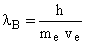and14
Here ao is the local Bohr radius, ve is the electron velocity inside the atom and h is the Planck constant.  In all these equations, the reference units are the ones existing in the frame where the atom is located.
This problem has also been explained previously (2), without all details.  These four principles must always be satisfied simultaneously.  The observer using the proper units, where the phenomenon takes place, needs to find the physical conditions (i.e. the electron velocity) so that all these principles are satisfied simultaneously.

5-- Method of Investigation.
Since we have to find a solution to the Bohr atom that must satisfy simultaneously the four fundamental equations above, we use a method consisting in a proposed solution that will evolve until the equations satisfying simultaneously the four fundamental principles can be found.  We calculate here the problem when an atom is transferred from location yo to a higher altitude y+Dy (figure 1).  First, we suggest a solution that will presumably satisfy all the four principles.  Then, this assumed solution will be tested, with all four physics principles above.
Proposed Solution-   We propose a solution with a constraint X-1, which will be tested below.  We have seen above (equation 9) that due to the principle of mass-energy conservation, the electron mass in the atom located in the frame at altitude y+Dy in the gravitational potential is: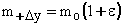15
We have demonstrated previously (2), that the physical length of matter (for example, the Bohr radius) decreases  (1+e) times when the electron mass increases (1+e) times.  This is in agreement with the Pound and Rebka's experiment (6).  Therefore the proposed solution is:
X-1   Due to the increase of gravitational potential when the particle is raised by +Dy, while the electron mass increases (1+e) times, the Bohr radius ro decreases (1+e) times. This gives: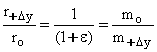16
Where r+Dy is the Bohr radius when the atom is at a location +Dy above the initial location. The sub index o refers to the initial gravitational frame of the atom, and the sub index +Dy always refers to an atom located at height +Dy above the original height yo in a gravitational potential.
In our solution, we do not propose any specific value of electron velocity in orbit around the nucleus.  The electron velocity with respect to the nucleus will be calculated below using the classical laws of physics. This unknown electron orbiting velocity in the upper frame in the gravitational field is represented by v+Dy.  We have vo is the normal orbiting electron velocity with respect to the nucleus in a non-distorted atom at the initial location yo in the gravitational potential.  We verify now, whether the assumed solution involving the proposal X-1, is compatible simultaneously with all the four fundamental principles above.

6-- Number of Units.
We must give a warning about a possible confusion between the quantities used in this problem.  We have seen above in sections 2, 3, and 4, that the length of a rod always located in the same frame, can be represented by different numbers, depending on the reference units used to measure it.  When we refer to "x meters" located in a given gravitational potential, it is impossible to distinguish whether we refer to the numerical "number x" (a pure number) times an assumed standard units of length, or if we refer to the "physical length" equal to "x meters".  Those are two different things.  The first one is a simple mathematical number while the second is a physical length.  A more accurate definition is necessary.
We know that a physical length is normally expressed as a pure number "x" of units when it is implied that the observer uses a universal system involving changeless reference units in all frames.  However, since we have seen that reference masses and reference lengths are changing when switching frames, the same rod remaining in the same frame, can also be expressed by a different number {(1+e) times} of units, when it is measured with a reference meter, which is (1+e) times shorter.  Within a frame, this "change of size of reference units" to express an absolute constant physical length, is a pure mathematical transformation, requiring a different number of units.
However, if a rod is carried with the observer, from a frame in a gravitational potential to another frame in a different gravitational potential, then we see that the local number of units does not change, but the real physical length of the rod has changed.  Consequently, a traveling observer finds that the number of local reference units is exactly the same, either in the original frame yo or in the frame in a different gravitational potential y+Dy, because both the rod and the observer's reference units get longer at the same time when switching frames.
In order to avoid this ambiguities between the "number of units" and the "physical length", we use a different notation when we need to refer specifically to the number of reference units rather than the physical quantity involved. The use of the “number” of units (instead of the physical length) is necessary, because - the fundamental equations of mechanics - are valid only when we use the “number” of proper units, rather than the real physical size of the body.   However, when we apply the principle of mass-energy conservation, we must consider the absolute amount of matter, which means taking into account both the number of units, times the size of the unit of mass involved.
Unfortunately, the usual equations in physics completely rely on an assumption of a universal reference unit, which is incorrectly assumed to remain constant in all frames.  This hypothesis is erroneous because it is not compatible with the principle of mass-energy conservation (2).  The parameters in a normal "mathematical equation" give nothing but the number of units independently of the fact that we must use the number of proper units in mechanics and the absolute mass in order to apply correctly the principle of mass-energy conservation.
When needed, the number of units (of the physical quantity) is represented here by #r in the case of length, #m in the case of mass, and #E in the case of the number of unit of energy.  We must note that in previous papers (2-7), the same number of units was instead represented by the notation: N-r, N-m and N-E.  The need for such a notation can be illustrated clearly when we have the same number of units of mass in different frames, (therefore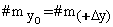).  In that case, we can see that although we have the same number of units, the physical amount of mass is different.

7-- Electron Velocity in Frame Y+Dy.
In order to test whether the proposed solution X-1 is compatible with all the physical principles, we need to calculate the electrical force F(e) between the electron e- and the proton p+ in a hydrogen atom in frame  y+Dy, at a distance +Dy above location yo, when calculated using the y+Dy units.   We have seen that the same classical physics relationship that exists in the frame yo, must also be valid in frame y+Dy.  The relationship giving the electrical force F(e) is:17
Equation 17 is valid only if we use the proper reference units existing in the frame where the mass is located.  However, that frame can be at any gravitational potential.  Therefore, we must calculate how all these physical quantities and also all the reference units are modified between the frame yo and y+Dy.  Let us write the (numerical) relationship existing in the yo frame.  When we are in the yo frame and we use the yo units, the Coulomb electrical force between the electron and the nucleus is:18
Equation 18 satisfies the behavior inside a normal atom at location yo before the atom is brought to a higher potential.  We first consider the same matter that was in the yo frame, which has been moved to the frame y+Dy.  It would be incoherent to use the physics relationship to predict the behavior of matter in frame y+Dy, using the yo units, because then, the result would not be compatible with the equations using the proper units at location y+Dy.
Let us apply the distortion corresponding to the proposed solution when the atom passes from location yo to y+Dy.  Then we must make use of the proposed solution X-1, (in section 5.1) requiring that the Bohr radius decreases (1+e) times (see equation 16).   At location y+Dy, this gives:19
Using a dimension analysis, it is possible to demonstrate that the size of the Coulomb constant ko is the same of both frames yo and y+Dy.  This will not be repeated here.  Equations 18 and 19 give: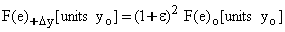20
Equation 20 shows the increase of Coulomb force between the electron and the proton is due to the reduction of the Bohr radius (when the atom is moved to the y+Dy frame), taking into account the suggested solution X-1.  This is calculated using the yo units, on both sides of the equation (i.e. the same size of units).  Equation 20 can be written: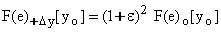21
We have seen above, in equation 12 (and in the note below equation 12), that Newton equation giving the electric force equals to the centrifugal force is valid only when we use the proper size of reference units where the phenomenon takes place.  Of course, this relationship is equivalent to considering only the number of proper units instead of the physical force.  Therefore the correct physical value must be calculated using the parameters in the y+Dy frame, where the particle is located.  Using that principle and equations 12 and 13, we get:22
Similarly to equation 22, in the yo frame, using yo units, the number of units of centrifugal force in a stable atom is:23
Equation 23 gives the relationship when the atom is in the yo frame.  That last relationship is not valid when the particle is in the +y+Dy frame.  We need equation 23 in order to calculate the physical changes taking place, when the atom passes from the yo to the +y+Dy frame.  We have seen in equation 21 that the force on the electron is (1+e)2 times larger in the y(+Dy) frame than when the atom is in the yo frame. Using the fundamental principles, equations 23, 22 and 21 give:24
However, we know that the number of units of mass and length is the same in all frames when we use local proper units. We have:25
Similarly, in the case of length, we have:26
Equations 25 and 26 in 24 give:27
We recall that the size of the reference unit "velocity" is the same in all frames.  This is obvious since a velocity is a length divided by time (i.e. difference of clock display) and that both length and time vary in the same proportion when moving to other frames.  Therefore the numbers of units of velocity is equal to the physical velocity, (since the size of the units are always the same).   Consequently, it is useless to specify the size of the [units].  Equation 21 gives:28
Equation 28 shows that in order to be compatible with the four fundamental principles above, with mass-energy conservation and also with the proposed solution X-1, the electron velocity in the frame y+Dy must be (1+e) times larger than in the yo frame.  Therefore when the atom passes from the frame yo to frame y(+Dy), the electron velocity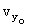inside the atom, around the proton, becomes (1+e) times faster.  We can see in the above calculation that nature behaves in such way that the physics, taking place in such a gravitational potential (at location y+Dy) is different from the one taking place at potential yo.  Of course, this is due to the increase of electron mass.  This is the only way to get matter compatible with the four above fundamental principles, as we will see below.  The problem of the orbiting electron around the nucleus is similar to the problem of Mercury around the Sun, in which there is an advance of the perihelion, so that the Newton equations are valid only when we use the units existing where the interaction takes place.  For the same reason, inside the atom, the electron velocity increases (1+e) times with respect to the initial frame, just as in the case of the larger velocity of Mercury around the Sun, which leads to the advance of the perihelion of Mercury.

8 -- Quantum Levels of Atoms in a Gravitational Potential.
Let us calculate the energy emitted by an atom after it has been moved to frame [y+Dy], as calculated by the observer, which uses [yo] units.  Using a dimension analysis, it can be demonstrated that the electric charge e- of the electron and the proton charge p+, are constants in any gravitational potential.  We have: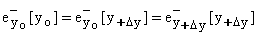29
A similar relationship also exists for the positive proton.  Furthermore, we have seen in equation 16 that the distance between the electron and the proton (the Bohr radius) decreases (1+e) times in the y+Dy frame.  Let us examine the electrostatic electron energy Ee between the electron and the proton of the atom, in this y+Dy frame.  Using the proper units in the initial frame, the electrostatic energy between the electron and the proton in the yo frame is:30
However, when the atom is in the y+Dy frame, we have an increase of gravitational potential so that the electron mass increases.  According to the proposed solution X-1 mentioned above, the Bohr radius ro decreases (1+e) times.  We recall that we will show below (in section 10) that this decrease of the Bohr radius is absolutely necessary to be compatible with the electron wavelength required in quantum mechanics.  Substituting this smaller radius, but using the yo units, the electrostatic electron energy Ee between the electron and the proton becomes:31
Equation 31 shows that, since the radius is smaller, the electrostatic electron energy Ee between the electron and the proton is (1+e) times larger after moving in the y+Dy frame.  This is compatible with the observations that an atom in a higher gravitational potential emits light at a higher frequency.  This "appears" as a blue shift of spectral lines.   The atomic energy levels of hydrogen, are shifted exactly as calculated and observed by Pound and Rebka (6).  Einstein's theory of relativity, which assumes a slowing down of “time” is illogical.  The clocks are just slowing down due to mass-energy conservation as shown here.
Coulomb Energy Curve. - On figure 2, we plot the Coulomb electron-proton energy curve for the atom in the frame yo and in the frame y+Dy.  Since the electric charge does not change between frames, the Coulomb curve is identical.  The level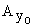represents the Lyman (n=1) electron orbit (8) when the atom is in its initial gravitational frame yo.  The levelrepresents the Balmer (n=2) term (8) of the Rydberg series, of the same quantum configuration, when the atom is in the same original frame yo.Figure 2
After the atom has been moved to the higherframe, the ground state of the atom is now shown at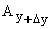and its corresponding Balmer series (n=2) is shown at.  Then, the size of the new Bohr radius has decreased (1+e) times.  Consequently, the size of all matter decreases also (1+e) times.  Figure 2 illustrates the physics taking place in an absolute frame, as seen by the observer in the initial yo frame.

9-- Planck Constant Relationship between Frames.
We demonstrate now that the size of the Planck constant unit is different in the y+Dy frame.  Of course, the number of units is the same in all frames.  When a mass is in the yo frame, and the observer uses the yo units, we have the relationship E=hn. Using the full notation this gives:32
We know also that the same equation E=hn is valid when the mass is in the y+Dy frame, when the observer is using the y+Dy units. The full notation gives:33

Also the (numerical value of) frequency measured by the yo observer is the same as the one measured by the y+Dy observer when the atom has moved from frame yo to frame y+Dy.  Of course, the absolute frequency emitted is higher when the atom is located in the y+Dy frame, but the local measuring clock also runs faster, so that the number frequency measured is the same.  This gives:34
From equation 15, we have seen that the electron mass increases (1+e) times when passing from the yo frame to the y+Dy frame.  Therefore the absolute size of the reference unit is (1+e) times larger in the y+Dy frame.  Since mass is the same physical phenomena as energy (differs only by c2), equation 15 gives:35
Equation 32 can be written: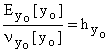36
Equation 33 can be written:37
Equation 35 and 34 in 37 gives: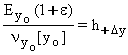38
Equations 36 and 38 give:39

From equations 39 and 34 we see that the size of the units of the Planck constant is (1+e) times larger in the y+Dy frame, while the number of units for the constant remains the same in all frames.

10-- Quantum Test using y+Dy Units.
Let us calculate the de Broglie electron wavelength measured by the observer using the y+Dy units.  We must substitute the local y+Dy parameters into the de Broglie equation.  Using equation 14 with the full notation in the yo frame, the de Broglie wavelength is:40
When the mass is in the y+Dy frame, using the yo units, the local observer finds:41
As calculated in equation 28, the electron velocity in the y+Dy frame is (1+e) times larger, with respect to the initial velocity in frame yo.  We found42
Also, when the atom moves to the y+Dy frame, its mass increases (1+e) times.  This gives: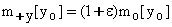43
Finally, when the mass moves to the y+Dy frame, we must substitute the new size of the Planck units in the y+Dy frame as given in equation 39.  The physical size of the Planck constant in the new frame is equal to the same number of units, times the size of the units, which is (1+e) times larger.  The substitution of these three equations (42, 43 and 39) in equation 41 gives:44
Equation 44 shows that the electron wavelength, and therefore the circumference of the Bohr orbit is (1+e) times smaller when the atom is in the y+Dy frame and measured with the yo units.  Therefore, this is a physical fact.  Let us now calculate the circumference of the Bohr orbit as measured by the observer using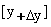units.
For the observer in the y+Dy frame, using the larger local mass reference units, the mass gives the same local number of units as for the yo observer.  In the case of the Planck parameter, even if the size of the reference unit is (1+e) times larger, the local number of units will also be the same number as for the yo observer.
Finally, since the size of the velocity reference unit is the same in both y+Dy and yo frames, the observer using the y+Dy units measures a number, which is (1+e) times larger.  However, since his local clock runs at a rate which is (1+e) times faster, he will measure the same frequency using his local second (of time), which is (1+e) times shorter.  This gives:45
Equation 45 shows that the observer, using the local units [y+Dy], measures that the circumference of the orbit is exactly equal to the de Broglie electron wavelength of the electron using the proper units [y+Dy], just as when the atom is in frame yo and using yo units.
One must conclude that the proposed solution satisfies, in the relevant proper frame, the conditions imposed by quantum mechanics.  Consequently, the atom measured by the moving observer "appears" perfectly identical to any atom at rest, observed by the rest observer.  However, it is clear that the absolute physical size of the atom at location [y+Dy] is (1+e) times smaller (Bohr radius) and (1+e) times more massive and with a faster orbiting electron.  But this change is undetectable by the [y+Dy] observer when using his local units.  Classical physics is still valid when using local units.  For the moving observer, these observations cannot inform him that he is actually at a higher gravitational potential.  This apparent normal atom measured by the observer in frame [y+Dy] will not appear normal for the observer in frame [yo], because it will not be compatible with the de Broglie equation.  The normal equation of mechanics are not valid when applied to an internal physical phenomenon taking place inside a foreign frame if it is at a different gravitational potential or has kinetic energy.  For the observer in frame [yo], the atom in frame [y+Dy]  will be physically (1+e) times smaller.  This explains logically the physical reality of the dilation and contraction of matter.  We see now how Einstein's relativity is illogical and useless.

11- Generalization of Potential and Gravitational Energy.
As a consequence of the principle of mass-energy conservation, we have seen above that, there is an internal rearrangement inside the atom.  The absolute physical parameters describing the atoms and molecules change due to the increase of electron mass.  In addition, we have seen that the atomic structure of matter is controlled by the de Broglie electron wavelength, which determines the size of the Bohr radius and the clock rate at which matter reacts in different frames.
We can show now that the properties of matter related to length and clock rates, can be described as a function of both kinetic and potential energy.  Knowing the kinetic energy and the gravitational potential energy of the atom, we can combine these two phenomena.  Let us calculate the relative change of de Broglie wavelength of the Bohr electron when the atom moves from rest to velocity va, at the time when the mass moves from location yo to location y+Dy in a gravitational potential.   We know that the circumference of the Bohr orbit must always be equal to the de Broglie wavelength using local units.  Always using yo units, in the original frame where the kinetic energy is zero at the gravitational location yo, the de Broglie wavelength is:46
When the atom moves to location y+Dy, and the atom remains stationary (zero velocity) in that new frame, equation 44 gives that the length of the circumference of the Bohr orbit is: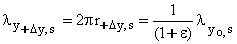47
Let us now consider the change of velocity in the new gravitational frame y+Dy.  From the paper: "Natural Length Contraction Due to Kinetic Energy" (5), we have seen in equation 28 (5), that at zero velocity, the electron wavelength in the Bohr orbit is: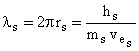48
In the same paper, equation 29(5) and 35(5) give:49
Equations 48 and 49 show that the de Broglie wavelength (Bohr orbit circumference) becomes g times larger when the velocity passes from zero to v.  This gives:50
Equation 50 shows that the de Broglie wavelength increases g times when the velocity increases to v.  Using the complete notation showing the particle in the gravitational frame at location y+Dy when the particle possesses velocity v gives, equations 50 applied to 47 gives:51
Equation 51 shows that when a mass acquires velocity (kinetic energy) inside a frame at the same time that it loses gravitational potential, equation 51 is applied.
When a mass is traveling freely (falling or traveling along an elliptical orbit) into a gravitational field we know that the increase of kinetic energy is equal to the decrease of potential energy.  Since there is no exchange of energy with the exterior of the system, there is no change of total energy with the interior.  Therefore, the electron mass remains constant.  However, in equation 51, we see that when a mass is falling freely into a gravitational field, the increase of kinetic energy makes g larger, while the decrease of gravitational potential makes the parameter (1+e) smaller.  Consequently, even if the electron mass remains constant during the fall of the atom, the overall effect is that the de Broglie electron length becomes larger.  Therefore, the size of the Bohr radius increases when a particle falls freely into a gravitational field, even if the electron mass remains constant.  From equation 51, when the particle travels freely at a variable velocity v at a variable distance Dy from a gravitational source, and the mass possesses the parameters (+Dy, v), the Bohr radius becomes:52
Naturally, the larger Bohr radius makes the clock run at a slower rate.  Consequently, since the Bohr radius is increasing during the free fall, and the behavior of matter depends on the parameters existing at the location and at the velocity matter is traveling, this is the fundamental mechanism which explains exactly the advance of the perihelion of Mercury in its elliptical orbit.  We see that when a planet (like Mercury) oscillates between perihelion and aphelion, the Bohr radius of all the atoms in the planet oscillates as given in equation 52.  Since the Bohr radius changes, the standard meter existing on the planet oscillates in length.  Furthermore, the local clock moving with Mercury acquires a different rate between perihelion and aphelion.  Since Newton's laws of physics must be applied using the units existing at the location where the matter is located, we see that the change of Bohr radius, which changes the local length and the local clock is responsible for the advance of the perihelion of Mercury as explained in more details in the paper: “ Detailed Classical Description of the Advance of the Perihelion of Mercury(3).  Consequently, the change of the electron mass and the change of the size of the Planck unit are not the only parameters responsible for the change of the Bohr radius.
All these explanations have been demonstrated using three-dimensional space, conventional logic, Newton's realistic physics, and a strict application of mass-energy conservation.  Einstein's general and special relativity is quite useless.

12 - Fundamental Nature of Kinetic Versus Potential Energy Interaction.
We have seen that due to the addition of potential energy to atoms, the mass of the particles increases and the Bohr radius decreases. However, when kinetic energy is added to atoms, the mass of the particles still increases, but the Bohr radius increases (5). Nevertheless, solutions in both frames are compatible with the principle of mass-energy conservation, classical physics, quantum mechanics and with all observational data.  Even if in both cases, the electron mass always increases, the increase of kinetic or potential energy produces an opposite change to the Bohr radius.  Let us examine the fundamental physical cause responsible for that behavior of the Bohr radius.  We can see that momentum conservation is involved.  We can see that energy acquired from gravitational potential possesses zero momentum, since the phenomenon is static.  However, in the case of kinetic energy, there is a momentum transfer to the electron since the energy must be in motion to transmit energy to a moving mass.  Let us examine how that difference of momentum transfer between potential and kinetic energy can produce a different effect on the electron structure of the atom.
Zero Momentum of Potential Energy. - When a body is raised, at zero velocity, from yo to a location y+Dy having a higher potential energy, the potential energy transmitted to the body does not possess any velocity.  Therefore, the increase of potential energy (which becomes new mass), which possess no momentum but must contributes to the increase of electron mass, needs to be accelerated to the velocity of the orbiting electron of an atom, in order to become absorbed in it.  This deficiency of momentum of the energy given to the atom slows down the electron velocity.  This problem of addition of potential energy (which is mass) having no velocity, to the moving orbiting electron is similar to the problem of the orbiting satellites around the Earth passing through stationary particles (gases) standing around the Earth.  It is well known that the drag produced by these stationary particles (hitting the moving satellite) slows down the velocity of the satellite, which produces a decrease of the altitude of the orbiting body, so that at a lower altitude, the satellite now moves at a higher velocity in a lower orbit. Similarly, this is what happens to the electron of an atom, which is slowed down (everywhere along the orbit) by the absence of momentum of the potential energy (absorbed by the moving electron), while the electron increases its mass.  Therefore, inside the atom, the radius of the electron orbit decreases as long as some energy (without momentum) is added to the orbiting electron.  This explains the shrinking of the Bohr radius calculated above, when there is an increased of gravitational energy which possesses no momentum.
Kinetic Energy Momentum. - When kinetic energy is added to atoms, then that energy (mass) possesses velocity and therefore also its own momentum.  Contrary to the case of potential energy, we can see that the kinetic energy transmitted to the mass possesses momentum, otherwise that force would not reach the atom which is already moving away.  Therefore the kinetic energy transmitted to the mass must possess momentum during the interaction.  Then, not only mass (implicated in the energy transfer), but also momentum is given up to the accelerated body and to the internal orbiting electrons.   We can see that the integrated momentum transferred to the orbiting electron produces a net effect on the orbiting electron.  Then, the addition of kinetic energy and momentum to the orbiting electron increases the size of the orbit, so that the centrifugal force around the nucleus increases and the radius of the Bohr orbit becomes larger.  This increase of momentum explains the increase of size of the Bohr orbit when the orbiting electron absorbs kinetic energy.  These considerations show the difference of the final atom structure (larger versus smaller Bohr radius) between an increase of potential energy, which does not possess any momentum and the increase of kinetic energy, which increases the size of the electron orbit.
This explains the increase of Bohr radius due to the kinetic energy and the decrease of Bohr radius due to the gravitational energy as calculated in this paper.  The complete calculation involving momentum conservation is beyond the scope of this paper.

13 - References
(1)  A. Einstein, Die Grundlage der allgemeine Relativitatstheorie, Ann. Phys. 49, 769-822 (1916).
(2) P. Marmet, Einstein's Theory of Relativity versus Classical Mechanics, Newton Physics Books, Ogilvie Road Gloucester On. Canada pp. 200,  ISBN 0-921272-18-9 (1997).  Also on the Web at: http://www.newtonphysics.on.ca/EINSTEIN/Chapter4.html
(3) P. Marmet,  Classical Description of the Advance of the Perihelion of Mercury", Physics Essays, Volume 12, No: 3, 1999, P. 468-487. Also, P. Marmet, A Logical and Understandable Explanation to the Advance of the Perihelion of Mercury", Society for Scientific Exploration, Albuquerque, June 3-5, 1999. Also on the Web: A Detailed Classical Description of the Advance of the Perihelion of Mercury.  At the address:
http://ww.newtonphysics.on.ca/MERCURY/Mercury.html
(4) P.Marmet, "GPS and the Illusion of Constant Light Speed" Galilean Electrodynamics, 2001.  Also in Acta Scientiarum, Universidade Estadual de Maringà, Maringà-Paranà-Brazil, Vol 22, 5,. page 1269-1279. Dec. 2000: The GPS and the Constant Velocity of Light.  Also presented at NPA Meeting University of Conn, Storrs,  June 2000.  On the Web at:
http://www.newtonphysics.on.ca/Illusion/index.html
(5) P. Marmet, "Natural Length Contraction Mechanism Due to Kinetic Energy"  J. New Energy 2001.  Also on the Web at: http://www.newtonphysics.on.ca/kinetic/length.html
(6) R. V. Pound and G. A. Rebka, Apparent Weight of Photons, Phys. Rev. Letters., 4, 337 1964.  Also R. V. Pound and J. L. Snider, Effect of Gravity on Nuclear Resonance Phys. Rev. B. 140, 788-803, 1965.
(7) P. Marmet, Explaining the Illusion of the Constant Velocity of Light, Meeting "Physical Interpretations of Relativity Theory VII" University of Sunderland, London U.K., 15-18, September 2000. Conference Proceedings "Physical Interpretations of Relativity Theory VII" p. 250-260 (Ed. M. C. Duffy, University of Sunderland).
(8) G. Herzberg,   Atomic Spectra and Atomic Structure,  Dover Publications, New York,  pp. 258.  1944.
-----------

-------------------------------------------------

Nov,  2001

Return to: Top of page

Return to: List of papers on the web
Information: About the author